Documentation

Waveform Segmentation Using Deep Learning

This example shows how to segment human electrocardiogram (ECG) signals using recurrent deep learning networks and time-frequency analysis.

Introduction

The electrical activity in the heart can be measured as a sequence of amplitudes away from a baseline signal. For a single normal heart beat cycle, the ECG signal can be divided into the following beat morphologies  :

• P wave — A small deflection before the QRS complex representing atrial depolarization

• QRS complex — Largest-amplitude portion of the heartbeat

• T wave — A small deflection after the QRS complex representing ventricular repolarization

The segmentation of these regions of ECG waveforms can provide the basis for measurements useful for assessing the overall health of the human heart and the presence of abnormalities . Manually annotating each region of the ECG signal can be a tedious and time-consuming task with the potential of being automated by signal processing and machine learning methods.

This example uses ECG signals from the publicly available QT Database  . The data consists of roughly 15 minutes of ECG recordings from a total of 105 patients. To obtain each recording, the examiners placed two electrodes on different locations on a patient's chest, resulting in a two-channel signal. The database provides signal region labels generated by an automated expert system . This example aims to use a deep learning solution to provide a label for every sample according to the region where the sample is located. This process of labelling regions of interest across a signal is often referred to as waveform segmentation.

To train a deep neural network to classify signal regions, you can use a Long Short-Term Memory (LSTM) network. This example shows how signal preprocessing techniques and time-frequency analysis can be used to improve LSTM segmentation performance. In particular, the example uses the Fourier synchrosqueezed transform to represent the nonstationary behavior of the ECG signal.

The first step is to download the data from the GitHub Repository. All browsers have a Downloads directory that can be identified through the Preferences. Locate the file QT_Database-master.zip in that directory and move it to a folder where you have write permission. This example assumes you have placed the file in your temporary directory, whose location is specified by MATLAB®'s tempdir command. If you have the data in a folder different from tempdir, change the directory name in the subsequent instructions. Start by unzipping the datafile.

unzip(fullfile(tempdir,'QT_Database-master.zip'),tempdir)

Unzipping creates the folder QT_Database-master in your temporary directory. This folder contains the text file README.md and the following files:

• QTData.mat

• Modified_physionet_data.txt

QTData.mat contains the data used in this example. The file Modified_physionet_data.txt provides the source attributions for the data and a description of the operations applied to each raw ECG recording.

QTData
QTData =
labeledSignalSet with properties:

Source: {105×1 cell}
NumMembers: 105
TimeInformation: "sampleRate"
SampleRate: 250
Labels: [105×2 table]
Description: ""

Use labelDefinitionsHierarchy to see a list of labels and sublabels.
Use setLabelValue to add data to the set.

QTData is a labeledSignalSet that holds the source ECG signals and the corresponding waveform labels together in a single object. The 105 two-channel ECG signals are contained in the Source property. The Labels property contains a table of waveform labels. Each channel was labeled independently by the automated expert system and is treated independently, for a total of 210 ECG signals. The waveform labels specify each sample of the signal as belonging to one of the following classes: P, QRS, T, and N/A. A value of N/A corresponds to samples outside of a P wave, a QRS complex, or a T wave.

Use the head command to inspect the first few rows of the table contained in the Labels property of QTData.

ans=8×2 table
WaveformLabels_Chan1    WaveformLabels_Chan2
____________________    ____________________

Member{1}      [225000×2 table]        [225000×2 table]
Member{2}      [225000×2 table]        [225000×2 table]
Member{3}      [225000×2 table]        [225000×2 table]
Member{4}      [225000×2 table]        [225000×2 table]
Member{5}      [225000×2 table]        [225000×2 table]
Member{6}      [225000×2 table]        [225000×2 table]
Member{7}      [225000×2 table]        [225000×2 table]
Member{8}      [225000×2 table]        [225000×2 table]

Each table row corresponds to a patient and each table column corresponds to a channel. Use the getSignal function to extract the signal data for the first patient. Use the getLabelValues function to extract labels for the first channel. Visualize the labels for the first 1000 samples using the displayWaveformLabels helper function.

patientID = 1;
signalVals = getSignal(QTData,patientID);
labelVals = getLabelValues(QTData,patientID,'WaveformLabels_Chan1');

displayWaveformLabels(signalVals(1,1:1000),labelVals.Value(1:1000))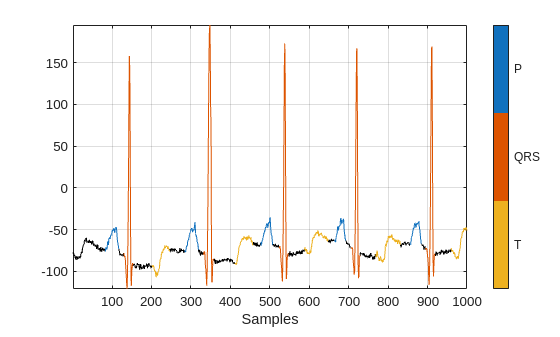Inspect the label values around the 150th sample, where the signal changes rapidly. The region marks the end of the QRS complex and the transition into N/A samples.

val = labelVals.Value(145:155)
val = 11×1 categorical array
QRS
QRS
QRS
QRS
QRS
QRS
QRS
QRS
n/a
n/a
n/a

The usual machine learning classification procedure is the following:

1. Divide the database into training and testing datasets.

2. Train the network using the training dataset.

3. Use the trained network to make predictions on the testing dataset.

The network is trained with 70% of the data and tested with the remaining 30%. To prevent any bias, no data belonging to the same patient is shared across the training set and testing set.

For reproducible results, reset the random number generator. Use the NumMembers property of the labeledSignalSet to extract the number of patients. Use the dividerand function to shuffle the patients and use the subset function to divide the data into training and testing labeledSignalSets.

rng default
[trainInd,~,testInd] = dividerand(QTData.NumMembers,0.7,0,0.3);

trainQT = subset(QTData,trainInd);
testQT = subset(QTData,testInd);

To input time-series data to the network, organize the data as cell arrays of matrices using the helper function resizeSignals. This helper function also divides the data into 5000-sample chunks to avoid excessive memory usage.

[signalsTrain,labelsTrain] = resizeSignals(trainQT);
[signalsTest,labelsTest] = resizeSignals(testQT);

Input Raw ECG Signals Directly into an LSTM Network

First, train the network using the raw ECG signals from the training dataset.

Define the network architecture before training. Specify a sequenceInputLayer of size 1 to accept one-dimensional time series. Specify an LSTM layer with the 'sequence' output mode to provide classification for each sample in the signal. Use 200 hidden nodes for optimal performance. Specify a fullyConnectedLayer with an output size of 4, one for each of the waveform classes. Add a softmaxLayer and a classificationLayer to output the estimated labels.

layers = [ ...
sequenceInputLayer(1)
lstmLayer(200,'OutputMode','sequence')
fullyConnectedLayer(4)
softmaxLayer
classificationLayer];

Choose options for the training process that ensure good network performance. Refer to the trainingOptions documentation for a description of each parameter.

options = trainingOptions('adam', ...
'MaxEpochs',10, ...
'MiniBatchSize',50, ...
'InitialLearnRate',0.01, ...
'LearnRateDropPeriod',3, ...
'LearnRateSchedule','piecewise', ...
'Plots','training-progress',...
'Verbose',0);

Train Network

Use the trainNetwork command to train the LSTM network. Due to the large size of the dataset, this process may take several minutes. If your machine has a GPU and Parallel Computing Toolbox™, then MATLAB automatically uses the GPU for training. Otherwise, it uses the CPU.

The training accuracy and loss subplots in the figure track the training progress across all iterations. Using the raw signal data, the network correctly classifies about 70% of the samples as belonging to a P wave, a QRS complex, a T wave, or N/A.

net = trainNetwork(signalsTrain,labelsTrain,layers,options);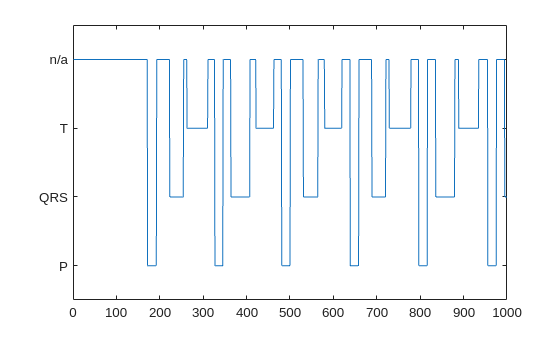Classify Testing Data

Classify the testing data using the trained LSTM network and the classify command. Specify a mini-batch size of 50 to match the training options.

predTest = classify(net,signalsTest,'MiniBatchSize',50);

A confusion matrix provides an intuitive and informative means to visualize classification performance. Use the confusionchart command to calculate the overall classification accuracy for the testing data predictions. For each input, convert the cell array of categorical labels to a row vector. Specify a column-normalized display to view results as percentages of samples for each class.

confusionchart([predTest{:}],[labelsTest{:}],'Normalization','column-normalized');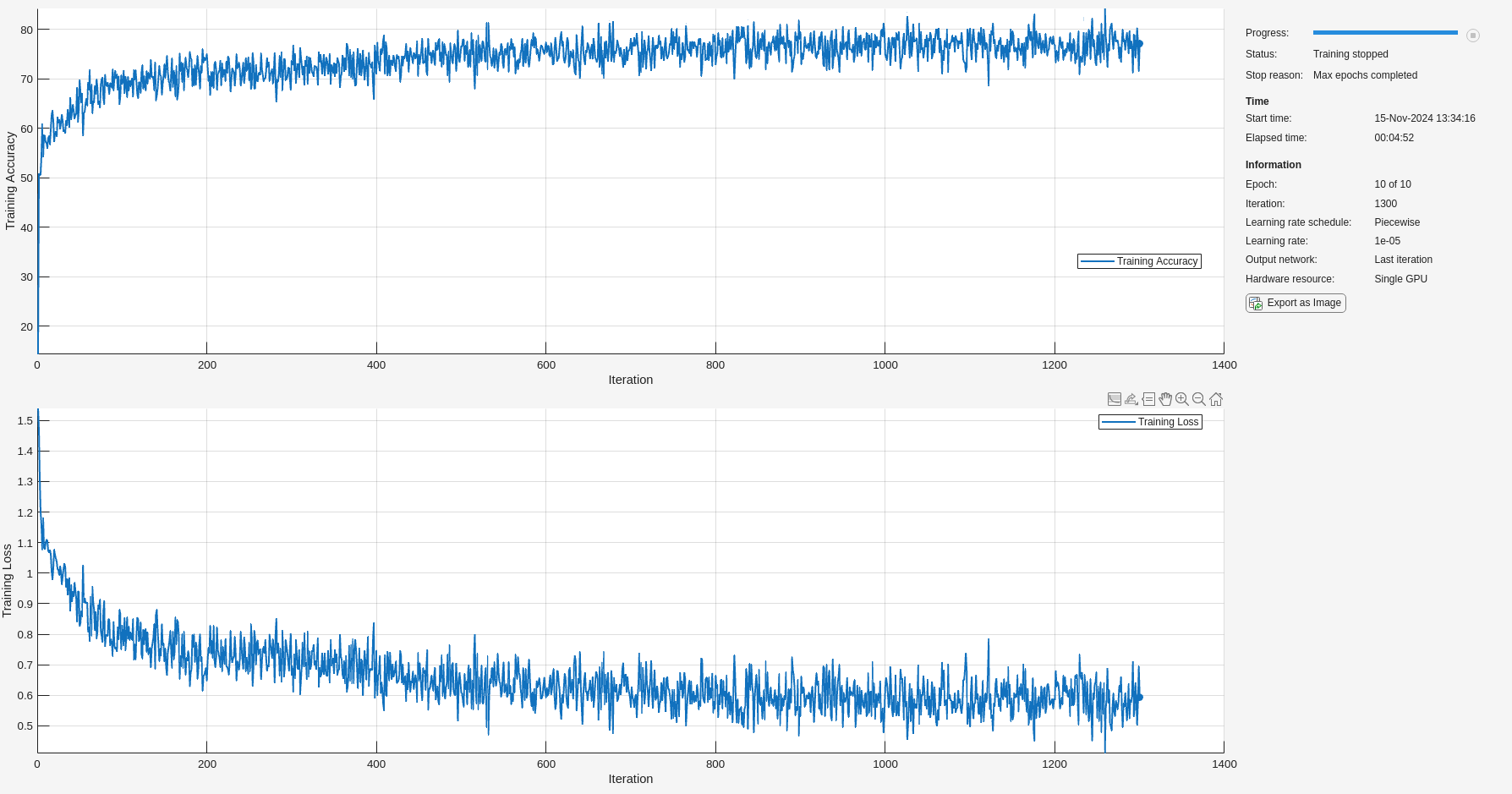Using the raw ECG signal as input to the network, only about 35% of P-wave samples, 60% of QRS-complex samples, and 60% of T-wave samples were correct. To improve performance, apply some knowledge of the ECG signal characteristics prior to input to the deep learning network, for instance the baseline wandering caused by a patient's respiratory motion.

Apply Filtering Methods to Remove Baseline Wander and High-Frequency Noise

The three beat morphologies occupy different frequency bands. The spectrum of the QRS complex typically has a center frequency around 10–25 Hz, and its components lie below 40 Hz. The P and T waves occur at even lower frequencies: P-wave components are below 20 Hz, and T-wave components are below 10 Hz .

Baseline wander is a low-frequency (< 0.5 Hz) oscillation caused by the patient's breathing motion. This oscillation is independent from the beat morphologies and does not provide meaningful information .

Design a bandpass filter with passband frequency range of [0.5, 40] Hz to remove the wander and any high frequency noise. Removing these components improves the LSTM training because the network does not learn irrelevant features.

Fs = QTData.SampleRate;
[~,dBP] = bandpass(signalsTrain{1},[0.5 40],Fs);

Specify an anonymous function, BPfun, to apply the bandpass filter to each signal.

BPfun = @(X) filter(dBP,X);

signalsFilteredTrain = cellfun(BPfun,signalsTrain,'UniformOutput',false);
signalsFilteredTest  = cellfun(BPfun,signalsTest,'UniformOutput',false);

Plot the raw and filtered signals for a typical case.

subplot(2,1,1)
plot(signalsTrain{210}(2001:3000))
title('Raw')
grid

subplot(2,1,2)
plot(signalsFilteredTrain{210}(2001:3000))
title('Filtered')
grid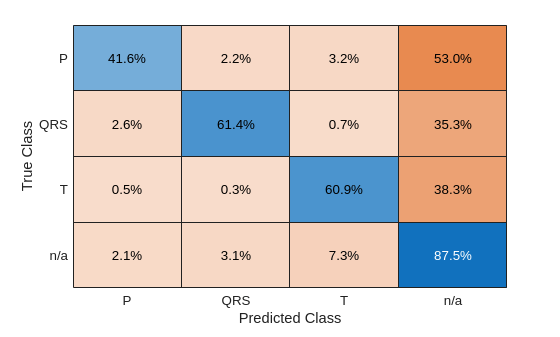Train Network with Filtered ECG Signals

Train the LSTM network on the filtered ECG signals using the same network architecture.

filteredNet = trainNetwork(signalsFilteredTrain,labelsTrain,layers,options);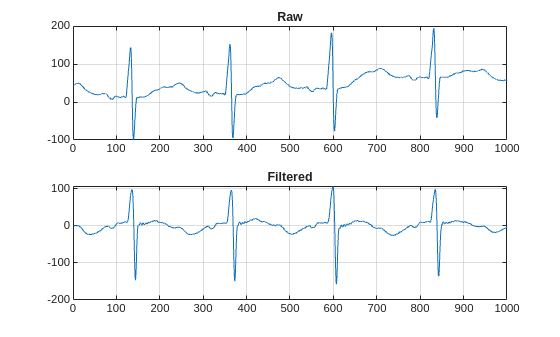Preprocessing the signals improves the training accuracy to better than 80%.

Classify Filtered ECG Signals

Classify the preprocessed test data with the updated LSTM network.

predFilteredTest = classify(filteredNet,signalsFilteredTest,'MiniBatchSize',50);

Visualize the classification performance as a confusion matrix.

figure
confusionchart([predFilteredTest{:}],[labelsTest{:}],'Normalization','column-normalized');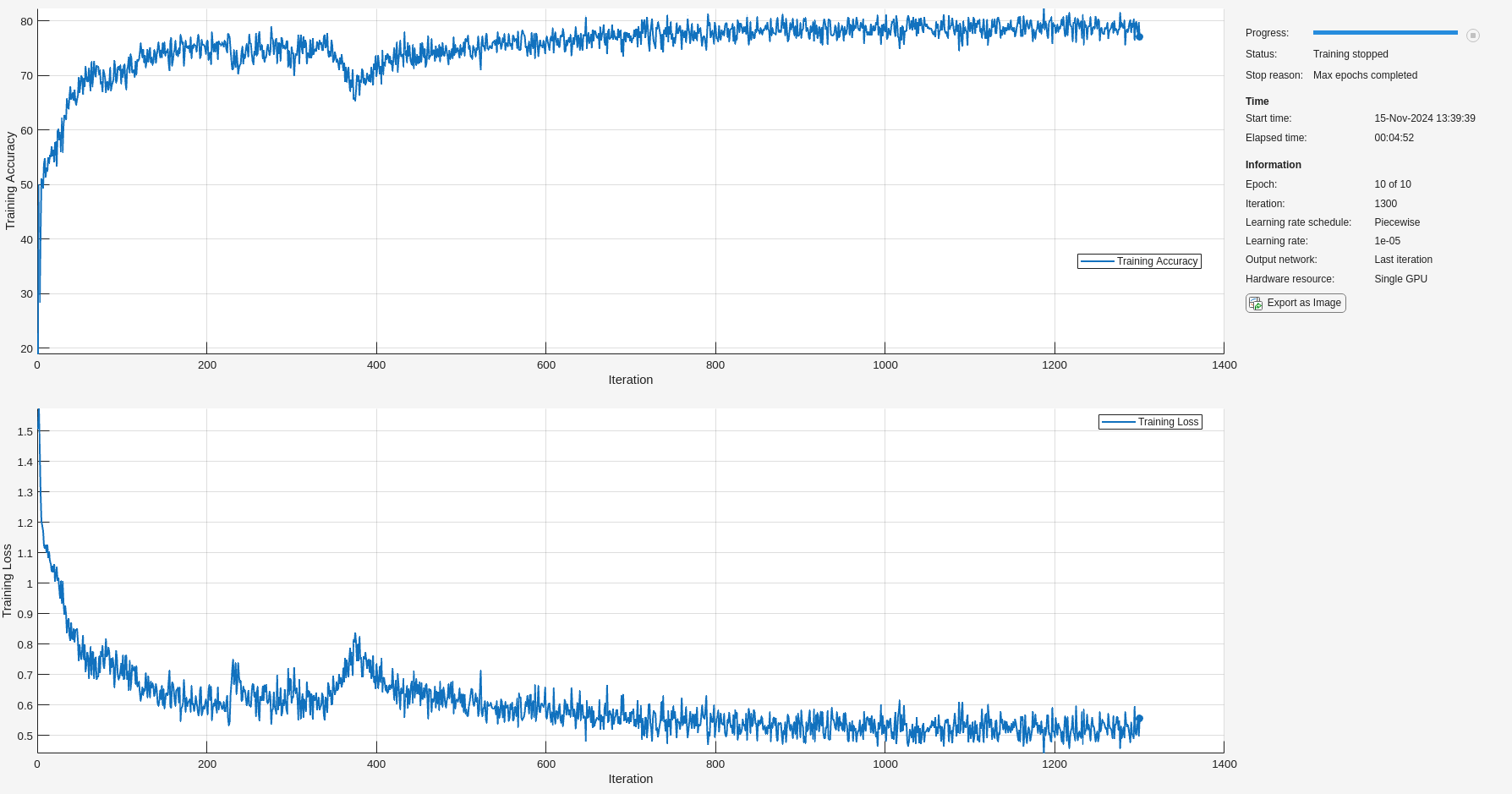Simple preprocessing improves P-wave classification by about 10%, QRS-complex classification by 10%, and T-wave classification by 20%.

Time-Frequency Representation of ECG Signals

A common approach for successful classification of time-series data is to extract time-frequency features and feed them to the network instead of the original data. The network then learns patterns across time and frequency simultaneously .

The Fourier synchrosqueezed transform (FSST) computes a frequency spectrum for each signal sample. Use the fsst function to inspect the transform of one of the training signals. Specify a Kaiser window of length 128 to provide adequate frequency resolution.

fsst(signalsTrain{1},Fs,kaiser(128),'yaxis')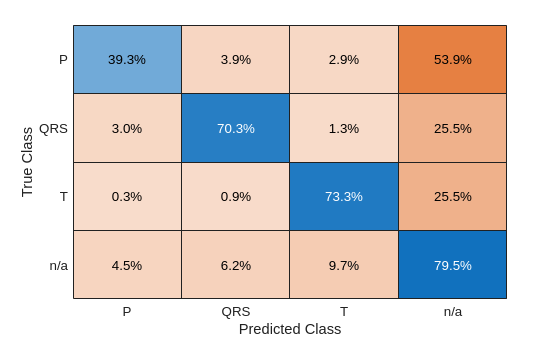Calculate the FSST of each signal in the training dataset. Extract the data over the frequency range of interest, [0.5, 40] Hz, by indexing the relevant content from the transform outputs. Treat the real and imaginary parts of the FSST as separate features and feed both components into the network.

Before training the network, standardize the training features by subtracting the mean and dividing by the standard deviation.

signalsFsstTrain = cell(size(signalsTrain));
meanTrain = cell(1,length(signalsTrain));
stdTrain = cell(1,length(signalsTrain));
for idx = 1:length(signalsTrain)
[s,f,t] = fsst(signalsTrain{idx},Fs,kaiser(128));

f_indices = (f > 0.5) & (f < 40);
signalsFsstTrain{idx}= [real(s(f_indices,:)); imag(s(f_indices,:))];

meanTrain{idx} = mean(signalsFsstTrain{idx},2);
stdTrain{idx} = std(signalsFsstTrain{idx},[],2);
end

standardizeFun = @(x) (x - mean(cell2mat(meanTrain),2))./mean(cell2mat(stdTrain),2);
signalsFsstTrain = cellfun(standardizeFun,signalsFsstTrain,'UniformOutput',false);

Repeat this procedure for the testing data. Standardize the testing features using the mean and standard deviation from the training data.

signalsFsstTest = cell(size(signalsTest));
for idx = 1:length(signalsTest)
[s,f,t] = fsst(signalsTest{idx},Fs,kaiser(128));

f_indices =  (f > 0.5) & (f < 40);
signalsFsstTest{idx}= [real(s(f_indices,:)); imag(s(f_indices,:))];
end

signalsFsstTest = cellfun(standardizeFun,signalsFsstTest,'UniformOutput',false);

Modify the LSTM architecture so that the network accepts a frequency spectrum for each sample instead of a single value. Inspect the size of the FSST to see the number of frequencies.

size(signalsFsstTrain{1})
ans = 1×2

40        5000

Specify a sequenceInputLayer of 40 input features. Keep the rest of the network parameters unchanged.

layers = [ ...
sequenceInputLayer(40)
lstmLayer(200,'OutputMode','sequence')
fullyConnectedLayer(4)
softmaxLayer
classificationLayer];

Train Network with FSST of ECG Signals

Train the updated LSTM network with the transformed dataset.

fsstNet = trainNetwork(signalsFsstTrain,labelsTrain,layers,options);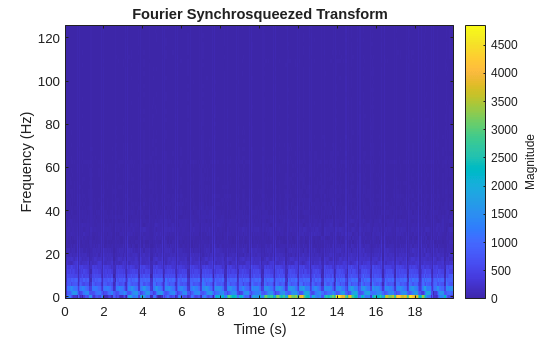Using time-frequency features improves the training accuracy, which now exceeds 90%.

Classify Test Data with FSST

Using the updated LSTM network and extracted FSST features, classify the testing data.

predFsstTest = classify(fsstNet,signalsFsstTest,'MiniBatchSize',50);

Visualize the classification performance as a confusion matrix.

confusionchart([predFsstTest{:}],[labelsTest{:}],'Normalization','column-normalized');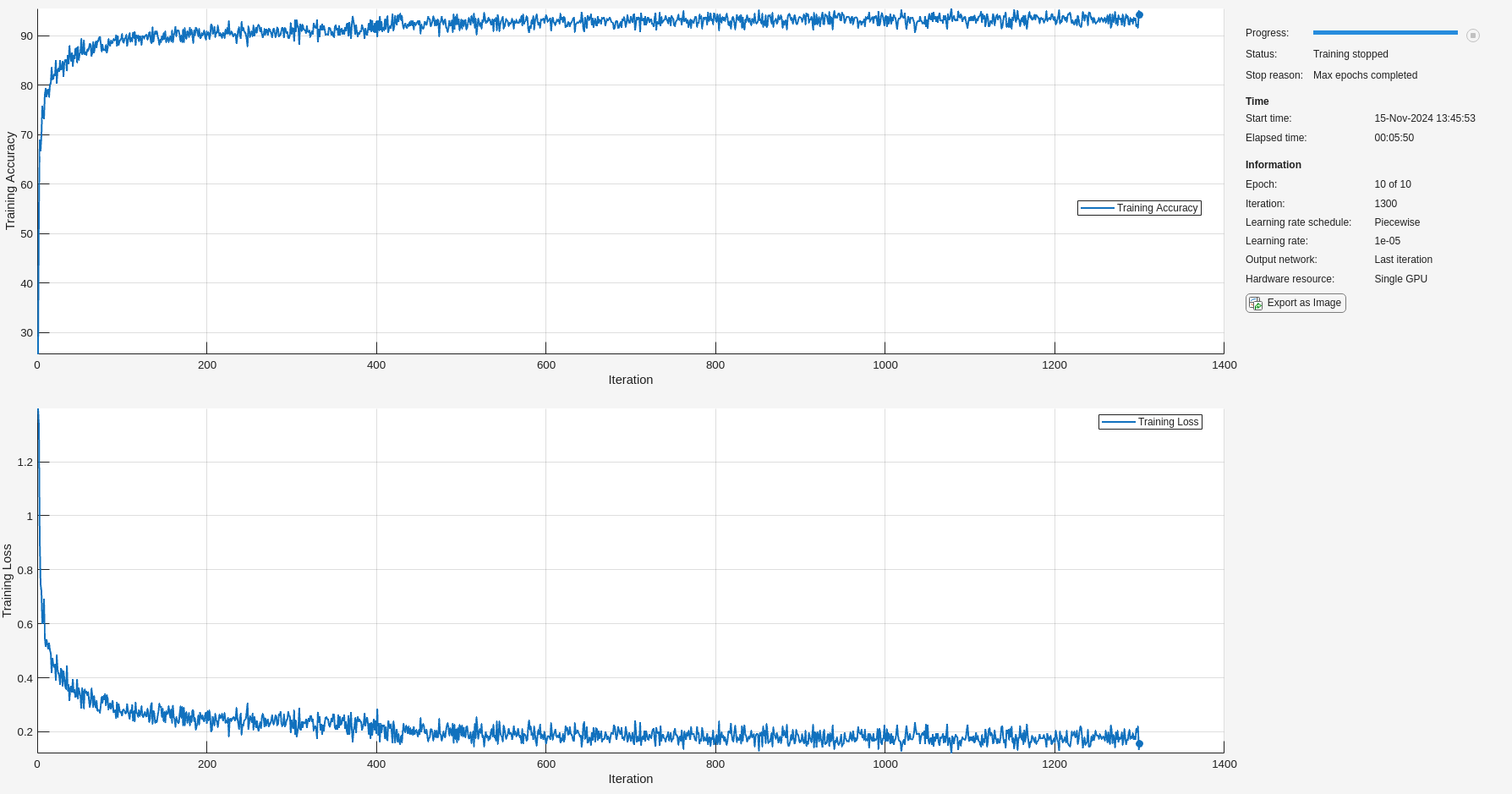Using a time-frequency representation improves P-wave classification by 50% (from about 35% to 85%), QRS-complex classification by 35%, and T-wave classification by 25%.

Use displayWaveformLabels to compare the network prediction to the ground truth labels for a single ECG signal.

subplot(2,1,1)
displayWaveformLabels(signalsTest{50}(1400:1900),labelsTest{50}(1400:1900))
title('Ground Truth')

subplot(2,1,2)
displayWaveformLabels(signalsTest{50}(1400:1900),predFsstTest{50}(1400:1900))
title('Predicted')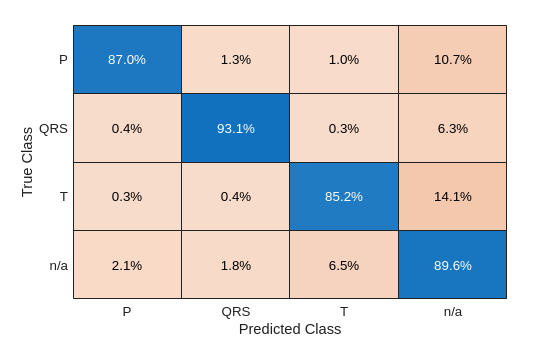Conclusion

This example showed how signal preprocessing and time-frequency analysis can improve LSTM waveform segmentation performance. Bandpass filtering and Fourier-based synchosqueezing result in an average improvement across all output classes from 60% to over 85%.

References

 McSharry, Patrick E., et al. "A dynamical model for generating synthetic electrocardiogram signals." IEEE® Transactions on Biomedical Engineering. Vol. 50, No. 3, 2003, pp. 289–294.

 Laguna, Pablo, Raimon Jané, and Pere Caminal. "Automatic detection of wave boundaries in multilead ECG signals: Validation with the CSE database." Computers and Biomedical Research. Vol. 27, No. 1, 1994, pp. 45–60.

 Goldberger, Ary L., Luis A. N. Amaral, Leon Glass, Jeffery M. Hausdorff, Plamen Ch. Ivanov, Roger G. Mark, Joseph E. Mietus, George B. Moody, Chung-Kang Peng, and H. Eugene Stanley. "PhysioBank, PhysioToolkit, and PhysioNet: Components of a New Research Resource for Complex Physiologic Signals." Circulation. Vol. 101, No. 23, 2000, pp. e215–e220. [Circulation Electronic Pages; http://circ.ahajournals.org/content/101/23/e215.full].

 Laguna, Pablo, Roger G. Mark, Ary L. Goldberger, and George B. Moody. "A Database for Evaluation of Algorithms for Measurement of QT and Other Waveform Intervals in the ECG." Computers in Cardiology. Vol.24, 1997, pp. 673–676.

 Sörnmo, Leif, and Pablo Laguna. "Electrocardiogram (ECG) signal processing." Wiley Encyclopedia of Biomedical Engineering, 2006.

 Kohler, B-U., Carsten Hennig, and Reinhold Orglmeister. "The principles of software QRS detection." IEEE Engineering in Medicine and Biology Magazine. Vol. 21, No. 1, 2002, pp. 42–57.

 Salamon, Justin, and Juan Pablo Bello. "Deep convolutional neural networks and data augmentation for environmental sound classification." IEEE Signal Processing Letters. Vol. 24, No. 3, 2017, pp. 279–283.